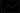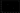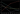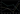# Python for machine learning, numpy and jupyter notebooks

27-Feb-2020
• machine learning
• engineering
3 min read / 751 words

In this series we'll go from (almost) zero to (almost) hero in Deep Learning, a field of machine learning. Very often the best way to learn is by doing!

In this post we'll install a Python 3 environment and start right away with Jupyter notebooks, a widely used interactive notebook where you can run python and all the libraries needed for machine learning. We won't go through a Python class here but if you're already familiar with programming, you should be able to follow along. If you need to learn Python first, there are many really good resources online. Same applies to basic linear algebra concepts like matrices, etc. If you want to download this post as a ready-made Jupyter notebook, here's the link.

#### Installing the environment

Miniconda is a self-contained Python environment. Install it from https://docs.conda.io/en/latest/miniconda.html

If you are on macOS Catalina (10.15), bear in mind that the default shell was changed from bash to zsh. So even after the Conda installation, it's likely that your terminal won't find the conda command. The easieast way to fix this is to revert back to the bash shell by going to Terminal -> Preferences and select Open shell with command: /bin/bash

#### Environment.yml

Now we'll set up the environment (loading the python libraries we'll need) by saving a file named environment.yml with the contents below:

`name: mlexerciseschannels:- defaultsdependencies:- python=3.6- bz2file==0.98- cython==0.28.*- flask==1.0.*- gensim==3.4.*- h5py==2.8.*- jupyter==1.0.*- matplotlib==2.2.*- numpy==1.14.*- pandas==0.23.*- pillow==5.2.*- pip==10.0.*- pytest==3.7.*- scikit-learn==0.19.*- scipy==1.1.*- seaborn==0.9.*- twisted==18.7.*- pip:  - PyHamcrest==1.9.0  - tensorflow==1.10.1  - keras==2.2.2  - jupyter_contrib_nbextensions==0.5.0  - tensorflow-serving-api==1.10.1`

Save it in a directory of your choice, that's where you'll start and save your jupyter notebooks.

Open the terminal and cd into that directory, then start the environment by executing

`conda env create`

Once the process ends, type

`conda activate mlexercises`

then

`jupyter notebook`

After the process ends, a browser window should open automatically and load jupyter at http://localhost:8888

#### The first jupyter notebook

Let's start with a new notebook then follow along with the code below! We'll begin by importing numpy, a very popular math library in machine learning, then create a few arrays, some basic math and concluding with some plots. Python code is shown here with a grey background, while output is shown as bulleted text.

`import numpy as np`
`a = np.array([1,3,2,4])`
`a`
• array([1, 3, 2, 4])
`type(a)`
• numpy.ndarray

This shows documentation in jupyter notebooks. Press esc to exit.

`a?`

Now let's create a matrix (2D array)

`b = np.array([[8, 5, 6, 1],              [4, 3, 0, 7],              [1, 3, 2, 9]])c = np.array([[[1, 2, 3],               [4, 3, 6]],              [[8, 5, 1],               [5, 2, 7]],              [[0, 4, 5],               [8, 9, 1]],              [[1, 2, 6],               [3, 7, 4]]])`
`b`
• array([[8, 5, 6, 1], [4, 3, 0, 7], [1, 3, 2, 9]])
`c`
• array([[[1, 2, 3], [4, 3, 6]], [[8, 5, 1], [5, 2, 7]], [[0, 4, 5], [8, 9, 1]], [[1, 2, 6], [3, 7, 4]]])

numpy arrays are objects. Press tab after the . to get a list of available methods.

Once you select a method from the list and open the parenthesis, press shift+tab+tab to get inline documentation.

The shape method tells us the dimension of the array. Python here tells us the object has four items along the first axis. The trailing comma is needed in Python to indicate that the purpose is a tuple with only one element.

If we write (4), this is interpreted as the number 4 surrounded by parentheses, and parentheses are ignored. Typing (4,) is interpreted as a tuple with a single element: the number 4. If the tuple contains more than one element, like (3, 4) we can omit the trailing comma.

`a.shape`
• (4,)
`b.shape`
• (3, 4)
`c.shape`
• (4, 2, 3)
`a`
• 1

Get the first subelement of the third element in c

`c[2,0]`
• array([0, 4, 5])

Get the first column in b

`b[:,0]`
• array([8, 4, 1])

The slice operator : will select a subset of an array.

`a[0:2]`
• array([1, 3])
`b[0:1, 0:1]`
• array([])

Here we select the second and third elements of a

`a[1:3]`
• array([3, 2])

and here from the second till the end of a

`a[1:]`
• array([3, 2, 4])
`and here from the beginning of a excluding the last item`
`a[:3]`
• array([1, 3, 2])

To cherry-pick items by spaced intervals we can use the following syntax. Here we select the first and third element in a

`a[0::2]`
• array([1, 2])

#### Some basic math

Math operators work element-wise.

`one = np.array([1,2])two = np.array([3,4])one + two`
• array([4, 6])
`3 * a`
• array([ 3, 9, 6, 12])
`a * b`
• array([[ 8, 15, 12, 4], [ 4, 9, 0, 28], [ 1, 9, 4, 36]])

#### Plotting

Here we'll use the Matplotlib library to generate graphs in order to visualize data. Visualization can have tremendous impact on debugging your dataset and analysing your models.

`import matplotlib.pyplot as plt`

After importing the library, we'll set some parameters that define how plots will look like:

`plt.style.use(['dark_background'])from matplotlib.pyplot import rcParamsfrom IPython.display import set_matplotlib_formatsrcParams['font.size'] = 14rcParams['lines.linewidth'] = 2rcParams['figure.figsize'] = (7.5, 5)rcParams['axes.titlepad'] = 14rcParams['savefig.pad_inches'] = 0.12set_matplotlib_formats('png', 'pdf')`

Let's plot our first array as a line chart:

`plt.plot(a)`For a scatter plot, we'll need to specify a symbol to plot.

`plt.plot(a, 'X')`Back to a line chart with the appropriate symbol.

`plt.plot(b, 'X-')`Feel free to go back to how we defined a and b to grasp the charts above.

`plt.plot(b.transpose(), 'X-')`Congrats for completing this introduction!